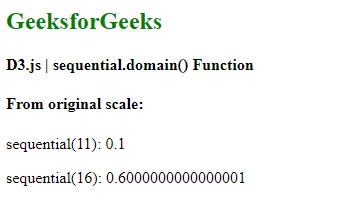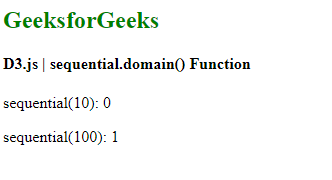# D3.js sequential.domain() Function

Sequential scales are very similar to continuous scale. On a continuous scale, mapping is done in a continuous way between domain and range. The only difference is that the output range of this scale is fixed by its interpolator and this range cannot be changed.

The sequential.domain() function is used to set the domain of the given sequential scale. The domain array always accepts two numeric values.

Syntax:

`sequential.domain([domain]);`

Parameters: This function accepts a single parameter as mentioned above and described below:

• domain: This sets the minimum and maximum domain value for the scale.

Return Values: This function does not return any value.

Example 1:

## HTML

 `  ` `<``html` `lang``=``"en"``>  ` ` `  `<``head``>  ` `    ``<``meta` `charset``=``"UTF-8"` `/>  ` `    ``<``meta` `name``=``"viewport"` `        ``path1tent="``width``=``device``-width,  ` `        ``initial-scale``=``1``.0"/>  ` ` `  `    ``<``script` `src``= ` `        ``"https://d3js.org/d3.v6.min.js"``> ` `    `` ` `  ` ` `  `<``body``>  ` `    ``<``h2` `style``=``"color:green"``> GeeksforGeeks ` `   `  `    ``<``h4``> D3.js | sequential.domain() Function ` `   `  `    ``<``script``>  ` `        ``var sequential = d3.scaleSequential() ` `            ``// Setting domain to [10,20] ` `            ``.domain([10,20]); ` ` `  `        ``document.write("<``h4``>From original scale:"); ` ` `  `        ``document.write("<``p``>sequential(11): ",  ` `                    ``sequential(11) + ""); ` ` `  `        ``document.write("<``p``>sequential(16): ",  ` `                    ``sequential(16) + ""); ` `    ``  ` ` ` ` `  ``

Output:Example 2: When a domain is given as string numbers it is parsed to number.

## HTML

 `  ` `<``html` `lang``=``"en"``>  ` ` `  `<``head``>  ` `    ``<``meta` `charset``=``"UTF-8"` `/>  ` `    ``<``meta` `name``=``"viewport"` `        ``path1tent="``width``=``device``-width,  ` `        ``initial-scale``=``1``.0"/>  ` ` `  `    ``<``script` `src``= ` `        ``"https://d3js.org/d3.v6.min.js"``> ` `    `` ` `  ` ` `  `<``body``>  ` `    ``<``h2` `style``=``"color:green"``> GeeksforGeeks ` `   `  `    ``<``h4``> D3.js | sequential.domain() Function ` `   `  `    ``<``script``>  ` `        ``var sequential = d3.scaleSequential() ` `            ``// Setting domain to ["10","100"] ` `            ``.domain(["10","100"]); ` ` `  `        ``document.write("<``p``>sequential(10): ",  ` `                    ``sequential(10) + ""); ` ` `  `        ``document.write("<``p``>sequential(100): ",  ` `                    ``sequential(100) + ""); ` `    ``  ` ` ` ` `  ``

Output:My Personal Notes arrow_drop_upCheck out this Author's contributed articles.

If you like GeeksforGeeks and would like to contribute, you can also write an article using contribute.geeksforgeeks.org or mail your article to contribute@geeksforgeeks.org. See your article appearing on the GeeksforGeeks main page and help other Geeks.

Please Improve this article if you find anything incorrect by clicking on the "Improve Article" button below.

Article Tags :

Be the First to upvote.

Please write to us at contribute@geeksforgeeks.org to report any issue with the above content.